Lesson 10: Acceleration

# Acceleration

Acceleration is a vector which measures the change in the velocity of an object.

• Don’t forget that velocity is a vector, so it has magnitude and direction.
• This means acceleration could be any of the following three…
1. a change in speed, the magnitude of the velocity (from 34 km/h to 67 km/h)
2. a change in direction (from East to North-East)
3. a change in both speed and direction (from 34 km/h East to 12 km/h West)

Acceleration is a measure of the rate at which velocity changes.

• Since velocity is a measure of the rate of change in displacement and had the equation…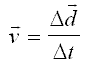the equation for acceleration should be similar...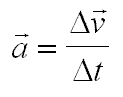a = acceleration (m/s/s or just m/s2)

Δv = change in velocity (m/s)

Δt = time interval (s)

Notice the units for acceleration.

• Since we take velocity and divide by time, we would get (without any simplification) m/s/s for units.
• What this really means is that for every second that passes the velocity of the object will change by this many metres per second.
• To make it a bit easier to read we use a little math to get m/s2 for the units.

Example 1: A car moving at 50km/h accelerates to 60km/h in 7.0 seconds. Determine its acceleration.

We first need to change the velocities from km/h into m/s:

50 km/h = 14 m/s

*N.B. keep the actual 13.88888889 that you see on your calculator for your calculations… never round off until the end of a problem!!!

60km/h = 17 m/s (same thing as the other velocity)

Now we use the formula, keeping in mind that Δv = vf - vi

a = Δv / Δt =(vf - vi) / t

= (17 - 14) / 7.0

a = 0.40 m/s2

If you got a different answer than this, check the following…

1. Did you use the rounded off values of 17m/s and 14 m/s, or did you use the actual values you saw on your calculator?
2. When you got to the end, did you use the rules for rounding off according to sig digs?

# Negative Acceleration

In physics, acceleration is not just an increase in velocity, but also a decrease in velocity.

• Even though you might have heard people use the word deceleration to describe an object slowing down, this isn’t really proper physics.
• Deceleration can only mean one thing.
• Instead, we call a decrease in velocity a negative acceleration.
• This actually helps us when doing calculations and also allows us to give more meaning to acceleration.

Example 2: You are walking down the street when you see an enormous, 112kg pickle rolling towards you at 12 m/s. You are, of course, surprised by a pickle of this size, let alone the fact that it is rolling down the street. You jump in front of it and begin pushing on it until you finally bring it to a stop 27.4s later. At this point you are arrested for interfering in the “World’s Largest Pickle Rolling Championships”. Determine the acceleration of the pickle.

In this example you have an object that is initially moving and ends up at rest. If you think of the Δv = vf - vi part of the acceleration formula, you’ll notice that a final velocity of zero (you stopped it), minus another number gives you a negative Δv. Since time is never negative, your acceleration will be negative.

a = Δv / Δt =(vf - vi) / t

= (0 - 12) / 27.4

a = -0.44 m/s2

In this case the negative sign means you were “taking away” velocity from an object that was moving in the positive direction to begin with. The object was slowing down.

Example 3: Once you have been arrested, the officials start rolling the pickle back towards the starting line so that Haans van der Winkle, the current champion from the Netherlands, can have a second try. After pushing for 8.8s, they get the pickle rolling backwards (towards the starting line) at 4.31m/s. Determine the acceleration of the pickle.

In this example, they cause an object to speed up, but it’s moving in the opposite direction. Since velocity is a vector, we can just put a minus sign in front of its speed and say that it is moving in the negative direction.

a = Δv / Δt =(vf - vi) / t

= (-4.31 - 0) / 8.8

a = -0.49 m/s2

In this case, does the negative acceleration mean the pickle was slowing down? Not at all! It was speeding up in the negative direction. A negative acceleration can mean…

1. An object moving in the positive direction (positive velocity) is slowing down.

or

2. An object moving in the negative direction (negative velocity) is speeding up.

So how do you keep track of all these types of acceleration. “Speeding up backwards means… I don’t know!”

• Try this. It always helped me.
• We usually visualize speeding up as positive, and slowing down as negative. I put that in the top row.
• A positive velocity means it’s going in the positive direction (like forwards), and a negative direction is backwards.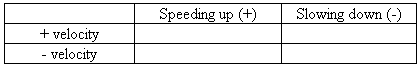• Now play a little “Battleship.”
• positive X positive = positive
• positive X negative = negative
• negative X negative = positive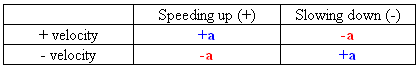• So, reading off the chart, an object moving backwards (-v) that is going faster and faster (speeding up) means it has a negative acceleration.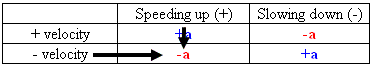• Go ahead and quickly sketch this out (from memory) on a piece of scrap paper at the start of an exam to help remind yourself!

# Change in Direction

Acceleration as a change in direction can be felt if you are in a car going through a turn.

• Even if it’s speed is the same (e.g. 50 km/h), the direction is changing
• You feel this acceleration as the you feel your body being “pushed” towards the outside of the curve.
• Your direction is changing at every instant!

This is why it is important to distinguish between “speed” and “velocity” and why we define acceleration as a change in velocity.

• Acceleration, like velocity, has direction.
• If we change either speed or direction or both, velocity changes and we accelerate.